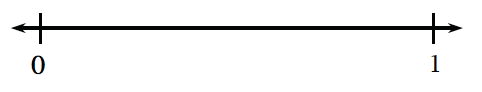### Home > ACC6 > Chapter 1 Unit 10 > Lesson CC2: 1.2.1 > Problem1-58

1-58.

Copy the number line below and place the following probabilities on it.1. A $\frac{1}{4}$ chance that you will be the team member who gets supplies tomorrow.

2. A $25\%$ chance of snow tomorrow.

3. A $0.8$ probability of eating vegetables with dinner.

4. P(blue marble) = $\frac { 5 } { 8 }$.

5. A $0.01$ probability that it will be $85^{\large \circ }$F on Saturday.

Convert every decimal and percent to a fraction. Review the Math Notes box in Lesson 1.1.5 or review your Toolkit entry for representations of portions.